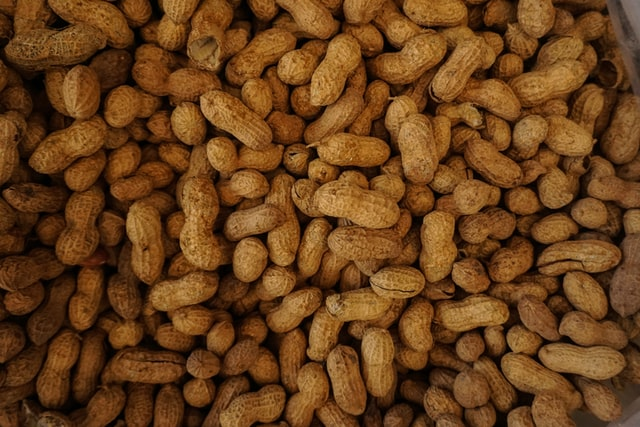## 22.6 Example: Cadmium in peanutsA study of peanuts from the United States found the sample mean cadmium concentration was 0.0768 ppm with a standard deviation of 0.0460 ppm, from a sample of size 290 peanuts gathered from a variety of regions at various times (attempting to find a representative sample).

The parameter is $$\mu$$, the population mean cadmium concentration in peanuts.

Every sample of $$n = 290$$ peanuts is likely to produce a different sample mean; that is, the sample means show sampling variation. The sampling variation can be measured using the standard error:

$\text{s.e.}(\bar{x}) = \frac{s}{\sqrt{n}} = \frac{0.0460}{\sqrt{290}} = 0.002701\text{ ppm}.$ The approximate 95% CI is $0.0768 \pm (2 \times 0.002701),$ or $$0.0768 \pm 0.00540$$, which is from 0.0714 to 0.0822 ppm. (The margin of error is 0.00540.)

If we repeatedly took samples of size 290 from this population, about 95% of the 95% CIs would contain the population mean (but this CI may or may not contain the value of $$\mu$$).

The plausible values of $$\mu$$ that could have produced $$\bar{x} = 0.0768$$ are between 0.0714 and 0.0822ppm. Alternatively, we are about 95% confident that the CI of 0.0714 to 0.0822 ppm straddles the population mean.

Since the sample size is larger than $$25$$, the CI is statistically valid.

### References

Blair BF, Lamb MC. Evaluating concentrations of pesticides and heavy metals in the U.S. Peanut crop in the presence of detection limits. Peanut Science. 2017;44:124–33.